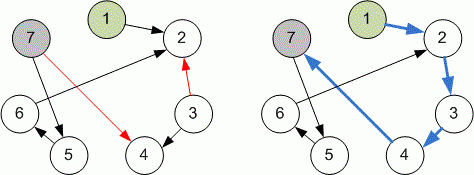Problems

# Reverse the graph

Time limit 1 second
Memory limit 128 MiB

You are given a directed graph with n vertices and m edges. The vertices in the graph are numbered from 1 to n. What is the minimum number of edges you need to reverse in order to have at least one path from vertex 1 to vertex n.

## Input data

First line contains two integers n and m\:(1 \le n, m \le 2 \cdot 10^6) — the number of vertices and the number of edges. The i-th line of the next m lines contains two integers x_i and y_i\:(1 \le x_i, y_i \le n), denoting that the i-th directed edge connects vertices from x_i to y_i.

## Output data

Print the minimum number of edges we need to invert. If there is no way of having at least one path from 1 to n, print -1.## Examples

Input example #1
7 7
1 2
3 2
3 4
7 4
6 2
5 6
7 5

Output example #1
2